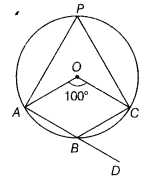# In the given figure, O is the centre of the circle. Then, find ∠CBD

In the given figure, O is the centre of the circle. Then, find ∠CBD.We know that the angle subtended by an arc at the centre is double the angle subtended by it at any point on the remaining part of the circle.
Then, ∠AOC=2∠APC
=>∠APC = 1/2 ∠AOC = 1/2 x 100° = 50°
Now, ∠APC + ∠ABC = 180°
[∵ sum of opposite angles of a cyclic quadrilateral is 180°]
=> 50° +∠ABC = 180°
=∠ABC = 180°- 50° = 130°
Also,∠ABC + ∠CBD = 180° [linear pair axiom]
=> 130° +∠CBD =180°
=>∠CBD = 180° - 130° = 50°